Want to share your content on R-bloggers? click here if you have a blog, or here if you don't.

15 years ago, a student of mine told me that I should start learning Python, that it was really a great language. Students started to learn it, but I kept postponing. A few years ago, I started also Python for Kids, which is really nice actually, with my son. That was nice, but not really challenging. A few weeks ago, I also started a crash course in Python, taught by Pierre. The truth is I think I will probably give up. I keep telling myself (1) I can do anything much faster in R (2) Python is not intuitive, especially when you’re used to practice R for almost 20 years… Last week, I also had to link Python and R for our pricing game : Ali wrote some template codes in Python, and I had to translate them in R. And it was difficult…

Anyway, since it was a school break this week, I said to my son that we should try to practice together, with a nice challenge. For those willing to try it, you’d better stop here, because I will spoil it.

The first page (so called “warming up”) is simple. In Python, use

```In  2 ** 38
Out 274877906944```

In R, it is also possible to do it

```2^38
 274877906944```

Then the idea is simple : in the url, change the 0 into 274877906944, then you will be redirected to the first page of the challenge….

Once you read the map.html page, you recognize Caesar cipher, and the hint is in the picture : K > M, O > Q and E > G. Ok, that’s an easy one, it is a translation of +2. The funny thing is that it was actually what we’ve seen in the previous course in Python ! So I tried to use the code I wrote that time :

```def cipher( text, key):
alphabet = "abcdefghijklmnopqrstuvwxyz"
crypted_text = ""
for c in text:
for i, l in enumerate(alphabet):
if c == l:
crypted_text += alphabet[(i+key)%26]
return crypted_text
```

When we tried, it worked well… but we get problems with spaces

```In  print(cipher("g fmnc wms bgblr",2))
Out ihopeyoudidnt```

Actually, I am not a big fan of the code in Python. While we’ve been seeing loops in our Python course, I tried my own code in R, to replicate

```cipher=function(phrase,k){
correspondance=data.frame(init=c(" ",letters),
fini=c(" ",letters[1+((k+0:25) %% 26)]))
phrase1=strsplit(phrase,"")[]
phrase2=NULL
for(i in 1:nchar(phrase)) phrase2=paste(phrase2,
as.character(correspondance[correspondance\$init
==phrase1[i],"fini"] ),sep="")
return(phrase2)
}```

which works here, since we got a sentence we can read

```cipher("g fmnc wms bgblr rpylqjyrc gr zw fylb. rfyrq ufyr amknsrcpq ypc dmp. bmgle gr gl zw fylb gq glcddgagclr ylb rfyr'q ufw rfgq rcvr gq qm jmle. sqgle qrpgle.kyicrpylq() gq pcamkkclbcb. lmu ynnjw ml rfc spj",2)
 "i hope you didnt translate it by hand thats what computers are for doing it in by hand is inefficient and thats why this text is so long using stringmaketrans is recommended now apply on the url"```

It says that we should use a Python function… but let’s keep playing with our R function. The hint is to use our cipher function on the url of the webpage

```cipher("map",2)
 "ocr"```

And indeed, we read the second step of the challenge on ocr.html. It says that we should look at the source of the page,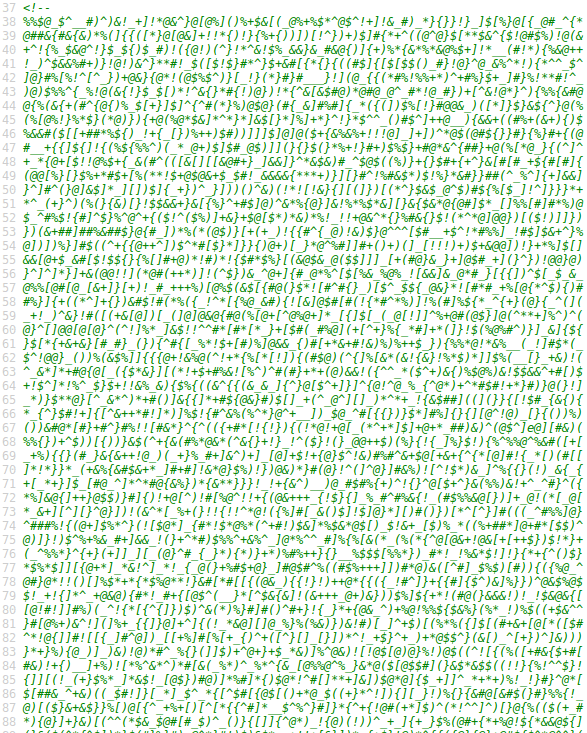That’s not too complicated, we should scan the page

```url="http://www.pythonchallenge.com/pc/def/ocr.html"
library(stringr)
L=scan("ocr.html",skip=37,n = 1256,what="character")
```

As said in the page, we should extract letters,

```C=NULL
for(i in 1:length(L)){
#L=scan("ocr.html",skip=i,n = 1,what="character")
LL=str_extract_all(L[i],"[a-zA-Z]")[]
if((length(LL)>0)){
cat(i,"....",LL,"\n")
C=paste(C,LL,sep="")}
}```

If we run it, we get the name of the next page,

```C
 "equality"```

On equality.html, it seems to be the same kind of game, except that here, we look for “One small letter, surrounded by EXACTLY three big bodyguards on each of its sides“. But the first step is to save that page and to scan it

```url="http://www.pythonchallenge.com/pc/def/equality.html"
library(stringr)
L=scan("equality.html",skip=21,n = 1250,what="character")
```

We need to find the proper code to seek regular expressions. My first idea was to use

`str_extract_all(L[i],"[A-Z]{3}[a-z]{1}[A-Z]{3}")[]`

but it did not work.. and indeed, if we have exactly three capital letters, we have to make sure that before and after, we do not have capital letters…

```C=NULL
for(i in 1:length(L)){
LL=str_extract_all(L[i],"[^A-Z]+[A-Z]{3}([a-z])[A-Z]{3}[^A-Z]+")[]
if((length(LL)>0)){
LL2=str_extract_all(LL,"[A-Z]{3}[a-z]{1}[A-Z]{3}")[]
LL4=substr(LL2,4,4)
cat(i,"....",LL4,".....",LL,"\n")
C=paste(C,LL4,sep="")}
}```

Here we get

```C

Here it’s a bit tricky.. the next page is not linkedlist.html but linkedlist.php. Again, look at the source of the page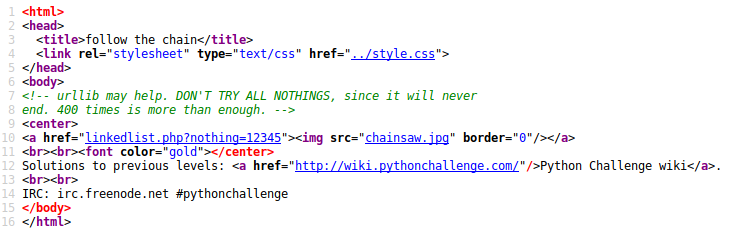it says to go to linkedlist.php?nothing=12345, and we have another locationOk… that can be long… so let’s loop. The idea is to seek for a number. If there is no number, we stop… if there are more than one number, we stop.

```NO=no=12345
i=1
continue=TRUE
while(continue){
i=i+1
L=scan(url,what="character")
L2=as.numeric(L)
if(sum(is.na(L2))!=(length(L)-1)) continue=FALSE
if(sum(is.na(L2))==(length(L)-1)){
cat(i,".......",no,"\n")
no=L2[!is.na(L2)]
NO=c(NO,no)
}
}```

We stop after 87 loops…

```no
 16044```

If we go on linkedlist.php?nothing=16044, we getso let us dived that number by two, and we continue

```no=no/2
NO=c(NO,no)
continue=TRUE
while(continue){
i=i+1
L=scan(url,what="character")
L2=as.numeric(L)
if(sum(is.na(L2))!=(length(L)-1)) continue=FALSE
if(sum(is.na(L2))==(length(L)-1)){
cat(i,".......",no,"\n")
no=L2[!is.na(L2)]
NO=c(NO,no)
}
}```

This time, the loop ends because we get two numbers

```L2
    NA    NA    NA    NA    NA 82683
    NA    NA    NA    NA    NA    NA    NA
 63579```

Let us look carefully at linkedlist.php?nothing=82682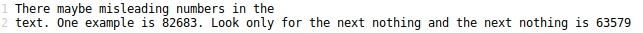so we should keep the second one

```no=L2[length(L2)]
NO=c(NO,no)
continue=TRUE
while(continue){
i=i+1
L=scan(url,what="character")
L2=as.numeric(L)
if(sum(is.na(L2))!=(length(L)-1)) continue=FALSE
if(sum(is.na(L2))==(length(L)-1)){
cat(i,".......",no,"\n")
no=L2[!is.na(L2)]
NO=c(NO,no)
}
}```

When it ends, we get

```no
 66831```

On linkedlist.php?nothing=66831, we get the name of the next page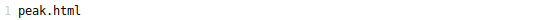Let us get on peak.html. Ok, that one is on peak hill – pickle – which is a Python function that I could not find on R…. let us skip it. Then we move to a channel.html page. Actually, it is necessary to lead a zip file

```download.file(url="www.pythonchallenge.com/pc/def/channel.zip",
destfile = "channel.zip" )
unzip("channel.zip",exdir = "./channel/")```

But that’s not enough… it is necessary to look at the comments in the zip file. It’s possible to create those comments when zipping via Python, but I could not see how to do it in R… Let us move to the hockey.html page, and to the oxygen.html page. And this one is fun.OK, there is this gray line. Somehow we should find the intensities of those gray boxes, and try to link those with letters/numbers.

```image="http://www.pythonchallenge.com/pc/def/oxygen.png"
library(pixmap)
library(png)
```

We can visualize those graph,

`image(t(IMG[,,2]))`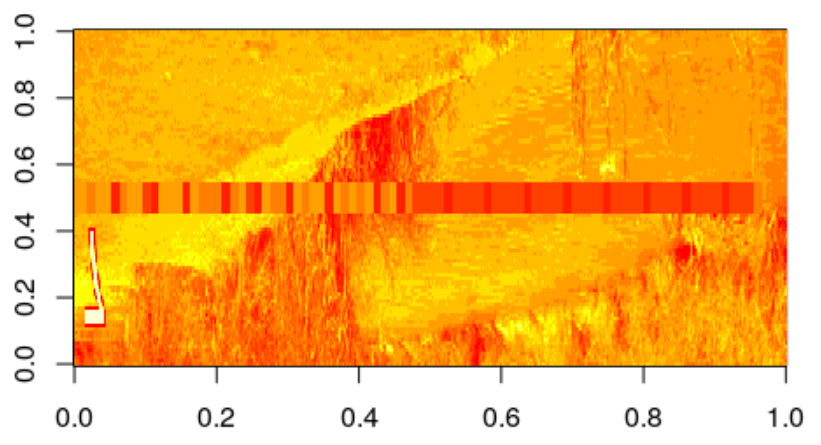`image(t(IMG[,,3]))`The grey line is one which remains unchanged in green and blue,

```j=45
L3=IMG[j,1:608,3]
L2=IMG[j,1:608,2]
prod(L2==L3)
 1```

Indeed, the two rows in the RGB decomposition are exactly the same here. Since a gray box is not on one pixel, we have to look for changes (and hope that there are no consecutive identical cells). Since here number are on a [0,1] scale, let us multiply by 255 (funny thing, we get integers).

```n=607
k=which(abs(L2[2:(n+1)]-L2[1:n])>.000001)
a=255*L2[c(k,n+1)]
range(a)
 32 121.```

Since the numbers are between 32 and 121 (128) we can look as if those are ASCII symbols,

```rawToChar(as.raw(a))
 "smart guy, you made it. the next level is [105, 10, 16, 101, 103, 14, 105, 16, 121]"```

Yesssss. Let us do it again here

```code=c(105, 10, 16, 101, 103, 14, 105, 16, 121)
rawToChar(as.raw(code))
 "i\n\020eg\016i\020y"```

Ok, for some reasons, I guess there is a problem here… let us add 100 if the numbers are smaller than 100,

```code[code<100]=100+code[code<100]
rawToChar(as.raw(code))
 "integrity"```

(this problem comes from the fact that I miss duplicated colors, i.e. numbers… so “11” becomes “1”, or more precisely, 110 is 10, 114 is 14, etc). And indeed, there is an integrity.html page. But let’s talk about it some other time…